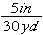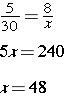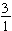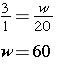# Scale Drawings and Scale Models

In earlier lessons, you learned about proportions and how to set them up and solve them. In this lesson, you will learn to use scale drawings and scale models to solve problems. A scale drawing shows an object with its measurements in proportion to the actual measurements of the object. They are similar (proportional) to the actual object. The scale is a ratio that compares the measurement used in a scale drawing with the actual measurements. Scales do not have to be in the same units. The scale factor is the constant of proportionality. When the units are the same, scale factor tells you how many times larger or smaller the actual object is compared to the measurements in the drawings or models.

Scale drawings are typically used when objects are too small or too large to be drawn to their actual size. Maps, house plans, and biology cell drawings are examples of items that use scale drawings. When using a model, scale factor is always the ratio of the model’s dimensions to the actual object’s dimensions.

## InvestigateThe Statue of Liberty is 1824 inches tall. Jamie bought a model in the souvenir shop that was 8 inches tall. How can we find out what scale factor was used to build the model?

Strategy

We know that the measurements of the statue and the model are proportional, so we can start by writing this as a proportion:.

Solution

We simplify the fraction by dividing 8 into both the numerator and the denominator to get. This means that the statue is 228 times taller than the model, or we reduced the size of the statue by 228 to get the model.

A map is a type of scale drawing. You can use the scale on a map to find real-world distances, or you can use the scale to make an accurate map.

## InvestigateWhat is the actual distance between the pond and the cabins?

Strategy

Write the scale as a ratio:Next, write a proportion and let x represent the actual distance.Solve the proportion:Solution

The actual distance between the cabins and the pond is 48 yards.

Scale Drawings are often used to build houses.

## InvestigatePatty’s father made her a dollhouse which was designed after the blueprint of their home. The blueprint is 20 inches wide by 42 inches long. The scale factor is. Find the size of the dollhouse.

Strategy

Think:Let the length be represented by l. Write a proportion.Solution

The dollhouse will be 60 inches wide and 126 inches long.

Here are some videos to help you along. Try to answer the questions along with the video, just like I hope you did with the questions above.

Video one – scale drawings

Video two – word problem

## Practice

1. The scale on a map is 5 centimeters = 75 kilometers. Two lakes on the map are located 8.1 cm apart. What is the actual distance between the two lakes?
2. The scale of a map is one and one quarter inches = 50 miles. On that map, two cities are four and one half inches apart. What is the actual distance between the cities?
3. Angela made a scale drawing of her bedroom using a scale of 0.5 cm = 1 meter. The actual length of her bedroom is 5 meters. What is the length of Angela’s bedroom on the scale drawing?
4. The Watson family went on vacation. On the first day, they drove a distance shown as 4 inches on a map. The actual distance that they drove was 240 miles. What was the scale of the map?

(source)